Next: Feedforward and backward computations Up: Model and learning algorithm Previous: Model and learning algorithm

## Improved approximation of the posterior probability

In , the posterior probability of the unknown variables was approximated as Gaussian distribution with diagonal covariance matrix. This means that the unknown variables were approximated to be independent given the observations. Notice that this assumption is false even if the unknown variables are assumed to be independent a priori. For instance, the factors are assumed to be independent a priori but observations induce dependencies between them. These dependencies are strongest for factors at the same time instant, that is, si(t) and sj(t) have a posterior dependence but si(t1)and sj(t2) can be nearly independent when t1 is far from t2.

The NLFA model has an indeterminacy of the rotation of the factors and the model can utilise this by choosing the rotation which makes the factors independent not only a priori but also a posteriori. However, the inclusion of the dynamic model causes posterior dependencies between factors at different time steps and these dependencies do not vanish for any rotation or any other mapping of the factor space. The smaller the process noise the stronger the dependence will be.

Taking into account the full posterior covariance between s(t-1) and s(t) is computationally costly if the dimension of the factor space is large. In practice, the most significant posterior correlations are the posterior autocorrelations of the factors, that is, the correlations between si(t-1) and si(t). They can be taken into account without increasing the computational complexity significantly.

In , the approximation of the posterior probability of the factors had the factorial form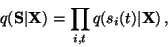(5)

where S denotes the factors and X the observations. The approximations q(si(t) | X) were Gaussian with mean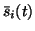and variance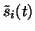. For the dynamical model introduced in this report, the approximation has the form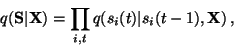(6)

where q(si(t) | si(t-1), X) is Gaussian and depends linearly on si(t-1):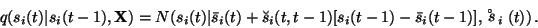(7)

Here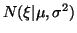denotes a Gaussian distribution over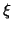with mean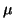and variance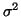.

Given si(t-1), the posterior variance of si(t) is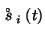and the posterior mean is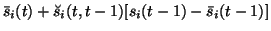. The approximate posterior q(si(t) | si(t-1), X) is thus parametrised by the mean, linear dependence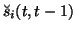and varianceas defined by (7), whereas in NLFA the posterior of the factor was parametrised by mean and variance alone.

It is easy to see by induction that if the past values of the factors are marginalised out, the posterior mean of si(t) isand posterior varianceis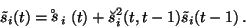(8)

Notice that the marginalised variancesare computed recursively in a sweep forward in time.Next: Feedforward and backward computations Up: Model and learning algorithm Previous: Model and learning algorithm
Harri Valpola
2000-10-17